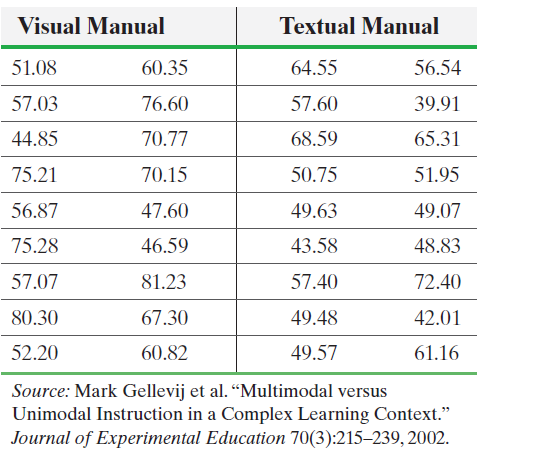×
Get Full Access to Statistics: Informed Decisions Using Data - 5 Edition - Chapter 11.3 - Problem 16
Get Full Access to Statistics: Informed Decisions Using Data - 5 Edition - Chapter 11.3 - Problem 16

×

# ?Visual versus Textual Learners Researchers wanted to know whether there was a difference in comprehension among students learning a computer program bISBN: 9780134133539 240

## Solution for problem 16 Chapter 11.3

Statistics: Informed Decisions Using Data | 5th Edition

• Textbook Solutions
• 2901 Step-by-step solutions solved by professors and subject experts
• Get 24/7 help from StudySoup virtual teaching assistantsStatistics: Informed Decisions Using Data | 5th Edition

4 5 1 271 Reviews
22
5
Problem 16

Visual versus Textual Learners Researchers wanted to know whether there was a difference in comprehension among students learning a computer program based on the style of the text. They randomly divided 36 students of similar educational level, age, and so on, into two groups of 18 each. Group 1 individuals learned the software using a visual manual (multimodal instruction), while group 2 individuals learned the software using a textual manual (unimodal instruction). The following data represent scores that the students received on an exam given to them after they studied from the manuals.(a) What type of experimental design is this?

(b) What are the treatments?

(c) A normal probability plot and boxplot indicate it is reasonable to use Welch’s t-test. Is there a difference in test scores at the= 0.05 level of significance?

Step-by-Step Solution:

Step 1 of 5) Visual versus Textual Learners Researchers wanted to know whether there was a difference in comprehension among students learning a computer program based on the style of the text. They randomly divided 36 students of similar educational level, age, and so on, into two groups of 18 each. Group 1 individuals learned the software using a visual manual (multimodal instruction), while group 2 individuals learned the software using a textual manual (unimodal instruction). The following data represent scores that the students received on an exam given to them after they studied from the manuals. (a) What type of experimental design is this (b) What are the treatments (c) A normal probability plot and boxplot indicate it is reasonable to use Welch’s t-test. Is there a difference in test scores at the = 0.05 level of significance

Step 2 of 3

Step 3 of 3

## Discover and learn what students are asking

Statistics: Informed Decisions Using Data : Estimating the Value of a Parameter
?What does the 95% represent in a 95% confidence interval?

Statistics: Informed Decisions Using Data : Inference about the Difference between Two Medians: Dependent Samples
?In Problems 3–10, use the Wilcoxon matched-pairs signedranks test to test the given hypotheses at the a = 0.05 level of significance. The dependent sa

Unlock Textbook Solution